# Understanding the "Option Strategy"

## 8.1 -Why to choose call over put?

The Bear call spread & the bear put spread are alike. It is a two-leg option strategy that is invoked when the market view is moderately bearish. In terms of payoff structure, the Bear Call Spread is very similar to the Bear Put Spread. However, there are some differences in strike selection and strategy execution. Bear Call Spread is a spread that uses 'Call options' instead of 'Put options', as in the bear put spread.

This stage may pose a fundamental question: Why should one choose a Bear Call spread instead of a Bear Put spread when they have similar payoffs?

This all depends on how attractive premiums are. The Bear Put spread can be executed with a debit while the Bear Call spread can be executed with a credit. If you're at a market point where -

1. Markets have rallied significantly (so CALL premiums are up).
2. Volatility is a positive
3. Sufficient time to the expiry.

If you are moderately bearish about the future, it is a good idea to invoke a Bear Call Spread to get a net credit instead of a Bear Put Spread to get a debit. Personally, I prefer strategies that offer net credit to strategies which offer a net debit.

## 8.2 - Few Strategy Notes

The Bear Call Spread, a traditional two-leg spread strategy that uses OTM Call options and ITM Call options, is the Bear Call Spread. You can also create the spread with other strikes. Remember that the spread (difference) between the selected strikes is a sign of profit potential.

To apply the bear call spread, -

1. Option 1: Buy 1 OTM Call
2. Option to sell 1 ITM call (leg 2)

Assure -

1. All strikes are part of the same underlying
2. The same expiry series
3. Each leg has the same number options

Let's take an example to better understand it.

Date: February 2016,

Outlook - Moderately bearish

Nifty Spot – 7222

1. Purchase 7400 CEYou can pay Rs.38/- premium. This is a debit transaction since money is being taken out of my account.
2. Buy 7100 CEYou will be rewarded with Rs.136/- in premium. Please note that this is an ITM option. This is a credit transaction since I get money.
3. The net cash flow (or the difference between debit and credit, i.e. 136 - 38) is the difference between debit and credit.+98Since this is a positive cashflow there is a net credit in my account.

A bear call spread generally has a net credit. This is why it is sometimes called a credit spread. The market can move in any direction after we have initiated the trade and it can expire at any level. Let's look at a few scenarios in order to see what the bear spread would do for different levels.

Scenario 1: Market expires at 7500 (above long Call).

Both the Call options would be worth 7500 and would therefore expire in the money.

• 7400 CE would have an intrinsic worth of 100. Since we paid a premium Rs.38, we would make a profit 100 - 38 = 662
• 7100 CE would have an intrinsic worth of 400. Since we sold this option at Ra.136 we would suffer a loss 400 - 13 =-264
• Net loss would be = -264 + 662- 202

Scenario 2: Market closes at 7400 (at long call).

The 7100 CE would lose its intrinsic value at 7400 and would therefore be worthless. The 7400 CE would be worthless.

• 7400 CE would become worthless and the entire premium of Rs.38 will be considered a loss.
• 7100 CE would have an intrinsic worth of 300. Since we sold this option at Ra.136 we would suffer a loss 300 - 13 =-164
• Net loss would be =-164 -38- 202

Note that the 7400 loss is the same as the 7500 loss. This is due to the fact the maximum loss above a certain point is 202.

Scenario 3: Market closes at 7198 (breakeven).

The trade is not profitable or loss-making at 7198. This is called a breakeven point. Let's see how this plays out.

• The 7100CE would be deemed dead at 7198 with an intrinsic value 98. We retain a part of the premium, i.e. 136 - 1998 =+38
• 7400 CE would be worthless and we will lose the premium, i.e 38
• Net payoff would be -38 + 38 = 0.

This shows that 7198 is not a profitable strategy.

Scenario 4: Market closes at 7100 (at a short call).

Both the Call options would cease to be worthless at 7100.

• 7400 would have no value and the premium would be worthless, i.e. Rs. 38
• 7100 will not also have an intrinsic value. Therefore, the entire premium paid i.e. Rs.136 would be retained.
• Net profit would be between 136 and 38 =98

The strategy is profitable as long as the market falls.

Scenario 5: Market expires at 7 000 (below the short-call)

This scenario shows how profitable the strategy is in the event that the market falls further. Both call options would be worthless at 7000. We will keep the 7400 CE premium, which is Rs. 38, as a loss. However, the 7100 CE premium (which is Rs.136) will be retained as a profit. The net profit of the strategy would therefore be 136-38 =98The strategy is clearly profitable when the market falls. However, it has a limit of Rs.98.

Here's the payoff at different expiries.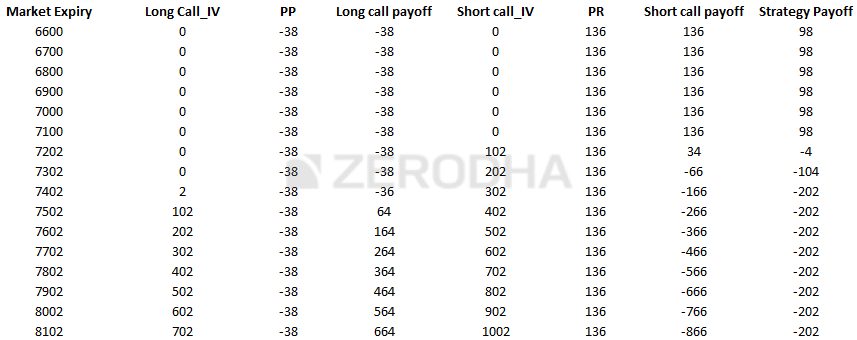You can plot these payoffs to see the graph of strategy payoff.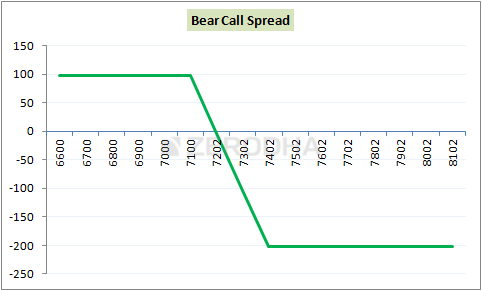In terms of of payoff, it's quite similar to the bear put spread where the scenario is predefined i.e . profit under best and losses under worst case.

## 8.3 - Strategy Generalization

The key trigger points of the strategy can be derived from the payoff.

• Spread = Difference between strikes
• 7400-7100 = 300
• 136 - 38 =98
• Breakeven = Lower strike + Net credit
• 7100 +98 = 7198
• Maximal Profit = Net Credit
• Max Loss = Spread + Net Credit
• 300 - 98 = 200

We can now add the Deltas together to calculate the overall delta for the strategy's sensitivity.

The following Delta values were derived from the BS calculator:

• 7400 CE is an OTM option with a delta of +0.32
• 7100 CE is an ITM option with a delta of +0.89
• We are short 7100 CE so the delta is -(+0.89) = 0.89
• The overall position delta = +0.32 + (-0.89)-0.57

The strategy's delta is negative. It indicates that the strategy loses money when the underpinning goes up and makes money when it goes down.

## 8.4 - Volatility Impact and Strike Selection

These images will help us determine the best call option strike, given the expiry date. The split of the time frame (1st and second half of the series) has been discussed many times before. I will only post the graphs as well as the summary table.

Strikes to choose when we're in the 1 st halves of the series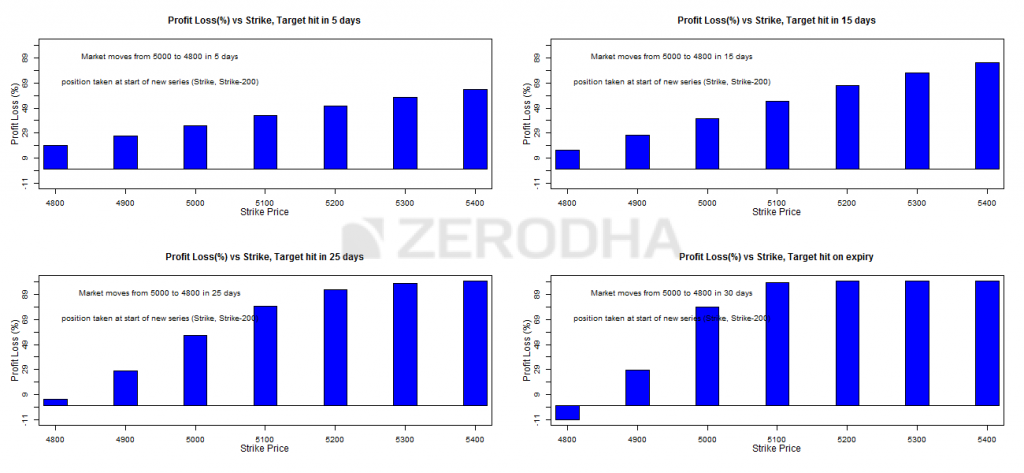.

4% move expected to happen within strike(higher)strike(lower)on graph
5 daysFar OTMATM+2 strikesTop to left
15 daysFar OTMATM + 2 strikesTop to  right
25 daysOTMATM + 1 strikeBottom to left
At expiryOTMATMBottom to right

Strikes to choose when we are in 2 nd the half of the series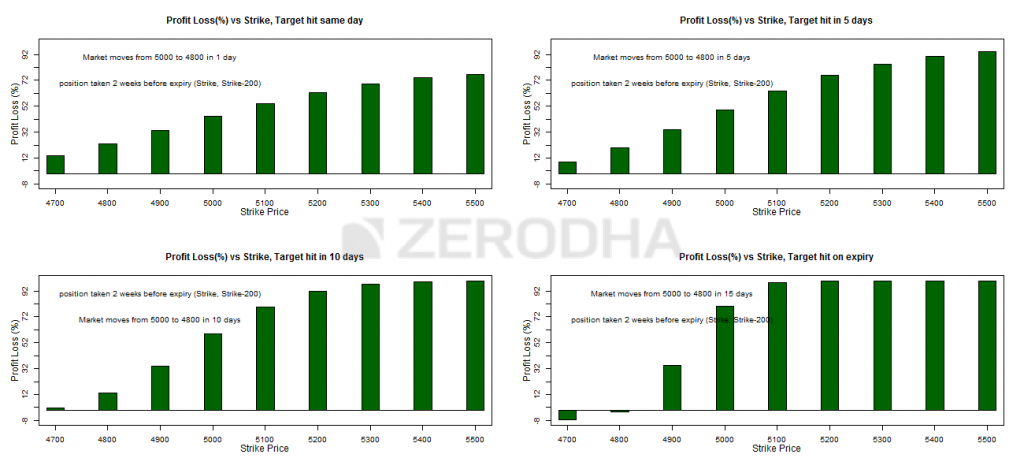4% move expected to happen within strike(higher)strike(lower)on graph
5 daysFar OTMFar OTMTop to  left
15 daysFar OTMSlightly OTMTop to right
25 daysSlightly OTMATMBottom to left
At expiryOTMATM/ITMBottom to right

The graph below shows the variation in strategy costs with respect to volatility changes.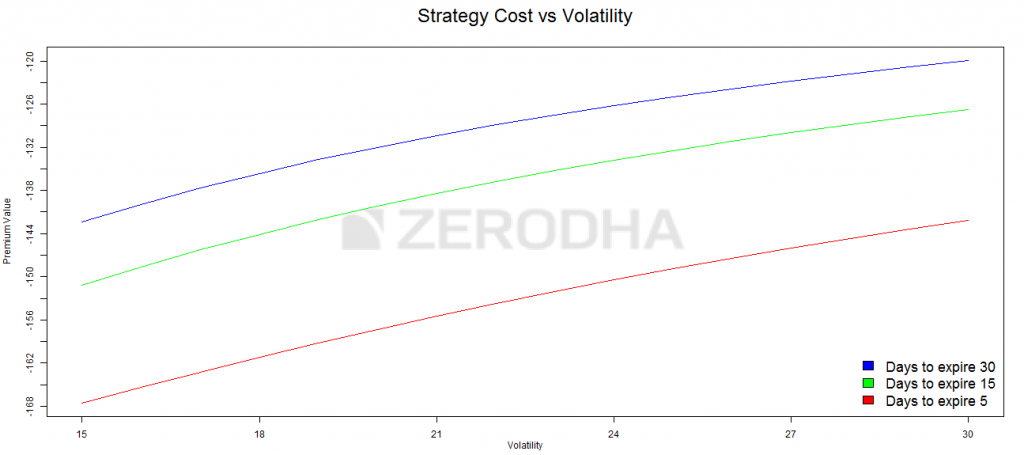The graph below shows how the premium changes with time and volatility.

• The blue line indicates that the strategy's cost is indicated by the blue line doesn't vary that much with volatility increasing, there is plenty of time for expiry(30 days)
• The green line indicates that the strategy's cost is high.variable with the rise in volatility, there is approximately have 15 days before the expiry date
• The red line indicates that the strategy's cost is high.Variations in this area can be significant with the rise in volatility, there is approximately five days before expiry.

These graphs show that volatility changes should not be worrying when there is plenty of time for them to expire. The volatility should be considered between the midpoint and the end of each series. If volatility is expected to rise, it is best to avoid taking the bear call spread.

### Keypoints

1. When you are moderately bearish about the markets, the bear call spread is most effective.
2. When call option premiums are greater than put options, you will choose a bear spread over a put spread.
3. Both profits and losses can be capped
4. Classic bear call spread includes simultaneously buying OTM call options, and selling ITM option calls
5. A bear call spread typically results in a net credit. This is another reason to invoke a Bear Call Spread versus a Bear Put Spread